Warning

This documents an unmaintained version of NetworkX. Please upgrade to a maintained version and see the current NetworkX documentation.

# average_shortest_path_length¶

average_shortest_path_length(G, weight=None)[source]

Return the average shortest path length.

The average shortest path length is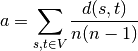where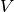is the set of nodes in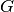,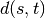is the shortest path from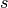to, and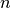is the number of nodes in.

Parameters: G (NetworkX graph) – weight (None or string, optional (default = None)) – If None, every edge has weight/distance/cost 1. If a string, use this edge attribute as the edge weight. Any edge attribute not present defaults to 1. NetworkXError – if the graph is not connected.

Examples

>>> G=nx.path_graph(5)
>>> print(nx.average_shortest_path_length(G))
2.0


For disconnected graphs you can compute the average shortest path length for each component: >>> G=nx.Graph([(1,2),(3,4)]) >>> for g in nx.connected_component_subgraphs(G): ... print(nx.average_shortest_path_length(g)) 1.0 1.0